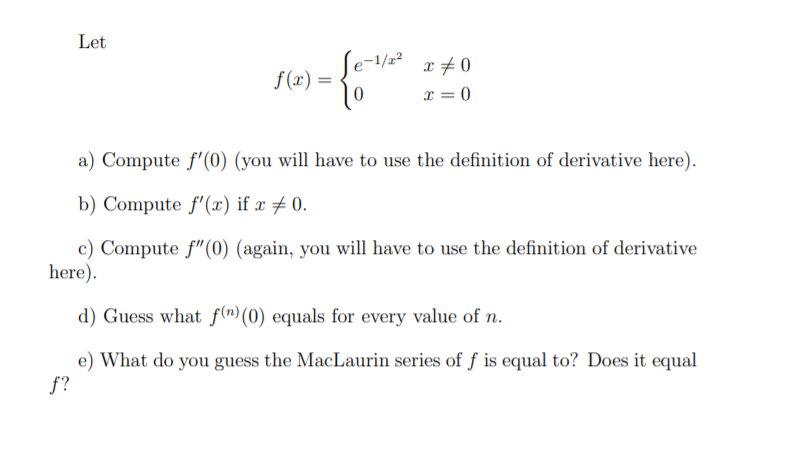# Let Se- f (x) = a) Compute f'(0) (you will have to use the definition of derivative here). b) Compute f'(æ) if x # 0. c) Compute f"(0) (again, you will have to use the definition of derivative here). d) Guess what f(n) (0) equals for every value of n. e) What do you guess the MacLaurin series of f is equal to? Does it equal f?

Question

calculus 2 picturehelp_outlineImage TranscriptioncloseLet Se- f (x) = a) Compute f'(0) (you will have to use the definition of derivative here). b) Compute f'(æ) if x # 0. c) Compute f"(0) (again, you will have to use the definition of derivative here). d) Guess what f(n) (0) equals for every value of n. e) What do you guess the MacLaurin series of f is equal to? Does it equal f? fullscreen